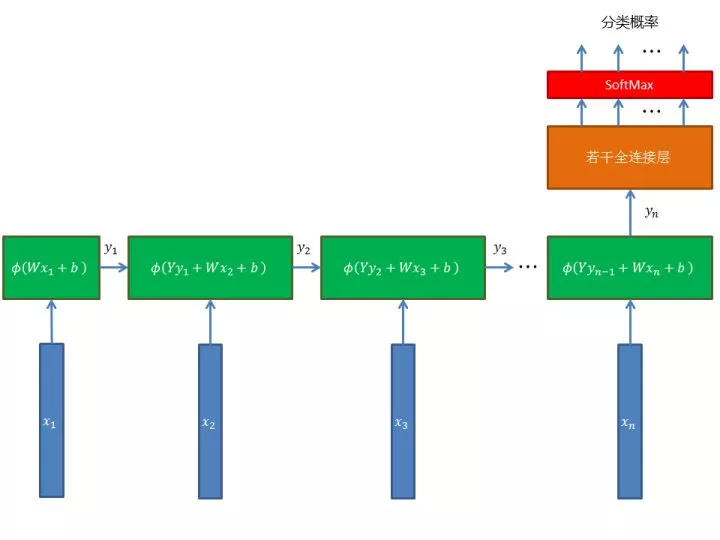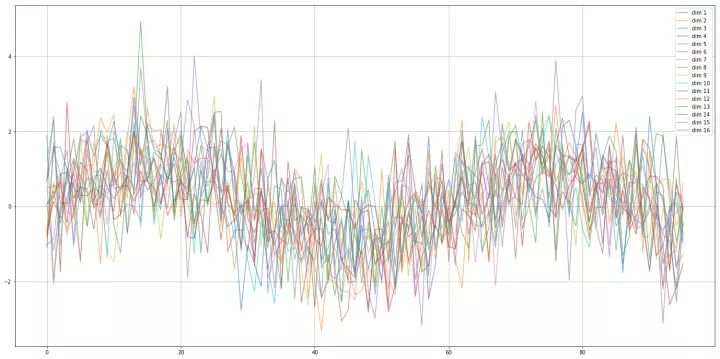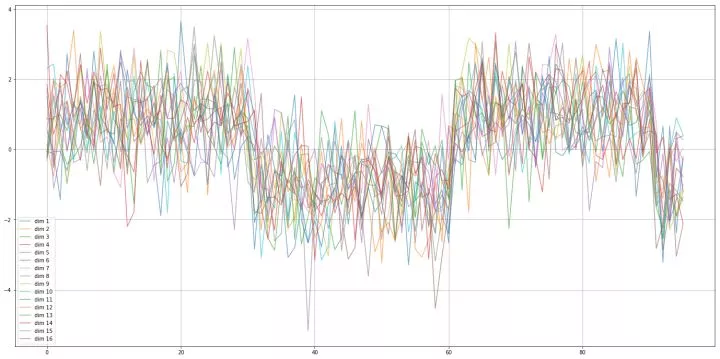# 运用计算图搭建递归神经网络（RNN）

• 2019-09-17
• 本文字数：5544 字

阅读完需：约 18 分钟## RNN

RNN 的思想是：网络也分步，每步以输入序列的该步数据（向量）和上一步数据（第一步没有）为输入，进行变换，得到这一步的输出（向量）。这样的话，序列的每一步就会对下一步产生影响。RNN 用变换的参数把握序列每一步之间的关系。最后一步的输出可以送给全连接层，最终用于分类或回归。RNN 有很多种，有一些复杂的变体，本文搭建一种最简单的 RNN ，它的结构是这样的：seq_len = 96  # 序列长度dimension = 16  # 序列每一步的向量维度hidden_dim = 12  # RNN 时间单元的输出维度# 时间序列变量，每一步一个 dimension 维向量（Variable 节点），保存在数组 input 中input_vectors = []for i in range(seq_len):    input_vectors.append(Variable(dim=(dimension, 1), init=False, trainable=False))    # 对于本步输入的权值矩阵W = Variable(dim=(hidden_dim, dimension), init=True, trainable=True)# 对于上步输入的权值矩阵Y = Variable(dim=(hidden_dim, hidden_dim), init=True, trainable=True)# 偏置向量b = Variable(dim=(hidden_dim, 1), init=True, trainable=True)# 构造 RNNlast_step = None  # 上一步的输出，第一步没有上一步，先将其置为 Nonefor iv in input_vectors:    y = Add(MatMul(W, iv), b)    if last_step is not None:        y = Add(MatMul(Y, last_step), y)    y = ReLU(y)    last_step = yfc1 = fc(y, hidden_dim, 6, "ReLU")  # 第一全连接层fc2 = fc(fc1, 6, 2, "None")  # 第二全连接层# 分类概率prob = SoftMax(fc2)# 训练标签label = Variable((2, 1), trainable=False)# 交叉熵损失loss = CrossEntropyWithSoftMax(fc2, label)

## 时间序列问题

def get_sequence_data(number_of_classes=2, dimension=10, length=10, number_of_examples=1000, train_set_ratio=0.7, seed=42):    """    生成两类序列数据。    """    xx = []    xx.append(np.sin(np.arange(0, 10, 10 / length)))  # 正弦波    xx.append(np.array(signal.square(np.arange(0, 10, 10 / length))))  # 方波    data = []    for i in range(number_of_classes):        x = xx[i]        for j in range(number_of_examples):            sequence = x + np.random.normal(0, 1.0, (dimension, len(x)))  # 加入高斯噪声            label = np.array([int(i == j) for j in range(number_of_classes)])            data.append(np.c_[sequence.reshape(1, -1), label.reshape(1, -1)])    # 把各个类别的样本合在一起    data = np.concatenate(data, axis=0)    # 随机打乱样本顺序    np.random.shuffle(data)    # 计算训练样本数量    train_set_size = int(number_of_examples * train_set_ratio)  # 训练集样本数量    # 将训练集和测试集、特征和标签分开    return (data[:train_set_size, :-number_of_classes],            data[:train_set_size, -number_of_classes:],            data[train_set_size:, :-number_of_classes],            data[train_set_size:, -number_of_classes:])

# 获取两类时间序列：正弦波和方波train_x, train_y, test_x, test_y = get_sequence_data(length=seq_len, dimension=dimension)## 训练

from sklearn.metrics import accuracy_scorefrom layer import *from node import *from optimizer import *seq_len = 96  # 序列长度dimension = 16  # 序列每一步的向量维度hidden_dim = 12  # RNN 时间单元的输出维度# 获取两类时间序列：正弦波和方波train_x, train_y, test_x, test_y = get_sequence_data(length=seq_len, dimension=dimension)# 时间序列变量，每一步一个 dimension 维向量（Variable 节点），保存在数组 input 中input_vectors = []for i in range(seq_len):    input_vectors.append(Variable(dim=(dimension, 1), init=False, trainable=False))    # 对于本步输入的权值矩阵W = Variable(dim=(hidden_dim, dimension), init=True, trainable=True)# 对于上步输入的权值矩阵Y = Variable(dim=(hidden_dim, hidden_dim), init=True, trainable=True)# 偏置向量b = Variable(dim=(hidden_dim, 1), init=True, trainable=True)# 构造 RNNlast_step = None  # 上一步的输出，第一步没有上一步，先将其置为 Nonefor iv in input_vectors:    y = Add(MatMul(W, iv), b)    if last_step is not None:        y = Add(MatMul(Y, last_step), y)    y = ReLU(y)    last_step = yfc1 = fc(y, hidden_dim, 6, "ReLU")  # 第一全连接层fc2 = fc(fc1, 6, 2, "None")  # 第二全连接层# 分类概率prob = SoftMax(fc2)# 训练标签label = Variable((2, 1), trainable=False)# 交叉熵损失loss = CrossEntropyWithSoftMax(fc2, label)# Adam 优化器optimizer = Adam(default_graph, loss, 0.005, batch_size=16)# 训练print("start training", flush=True)for e in range(10):    for i in range(len(train_x)):        x = np.mat(train_x[i, :]).reshape(dimension, seq_len)        for j in range(seq_len):            input_vectors[j].set_value(x[:, j])        label.set_value(np.mat(train_y[i, :]).T)        # 执行一步优化        optimizer.one_step()        if i > 1 and (i + 1) % 100 == 0:            # 在测试集上评估模型正确率            probs = []            losses = []            for j in range(len(test_x)):                # x = test_x[j, :].reshape(dimension, seq_len)                x = np.mat(test_x[j, :]).reshape(dimension, seq_len)                for k in range(seq_len):                    input_vectors[k].set_value(x[:, k])                label.set_value(np.mat(test_y[j, :]).T)                # 前向传播计算概率                prob.forward()                probs.append(prob.value.A1)                # 计算损失值                loss.forward()                losses.append(loss.value[0, 0])                # print("test instance: {:d}".format(j))            # 取概率最大的类别为预测类别            pred = np.argmax(np.array(probs), axis=1)            truth = np.argmax(test_y, axis=1)            accuracy = accuracy_score(truth, pred)            default_graph.draw()            print("epoch: {:d}, iter: {:d}, loss: {:.3f}, accuracy: {:.2f}%".format(e + 1, i + 1, np.mean(losses),                                                                                    accuracy * 100), flush=True)

epoch: 1, iter: 100, loss: 0.693, accuracy: 51.08%epoch: 1, iter: 200, loss: 0.692, accuracy: 51.08%epoch: 1, iter: 300, loss: 0.677, accuracy: 78.31%epoch: 1, iter: 400, loss: 0.573, accuracy: 49.31%epoch: 1, iter: 500, loss: 0.520, accuracy: 53.92%epoch: 1, iter: 600, loss: 0.599, accuracy: 97.08%epoch: 1, iter: 700, loss: 0.617, accuracy: 99.00%epoch: 2, iter: 100, loss: 0.601, accuracy: 94.46%epoch: 2, iter: 200, loss: 0.579, accuracy: 82.08%epoch: 2, iter: 300, loss: 0.558, accuracy: 76.15%epoch: 2, iter: 400, loss: 0.531, accuracy: 67.85%epoch: 2, iter: 500, loss: 0.507, accuracy: 63.77%epoch: 2, iter: 600, loss: 0.493, accuracy: 61.15%epoch: 2, iter: 700, loss: 0.479, accuracy: 62.23%epoch: 3, iter: 100, loss: 0.443, accuracy: 69.92%epoch: 3, iter: 200, loss: 0.393, accuracy: 85.85%epoch: 3, iter: 300, loss: 0.365, accuracy: 97.69%epoch: 3, iter: 400, loss: 0.284, accuracy: 95.08%epoch: 3, iter: 500, loss: 0.199, accuracy: 95.69%epoch: 3, iter: 600, loss: 0.490, accuracy: 80.62%epoch: 3, iter: 700, loss: 0.264, accuracy: 94.31%epoch: 4, iter: 100, loss: 0.320, accuracy: 83.46%epoch: 4, iter: 200, loss: 0.333, accuracy: 80.92%epoch: 4, iter: 300, loss: 0.276, accuracy: 90.15%epoch: 4, iter: 400, loss: 0.242, accuracy: 95.00%epoch: 4, iter: 500, loss: 0.217, accuracy: 96.38%epoch: 4, iter: 600, loss: 0.191, accuracy: 95.31%epoch: 4, iter: 700, loss: 0.167, accuracy: 94.00%epoch: 5, iter: 100, loss: 0.142, accuracy: 94.62%epoch: 5, iter: 200, loss: 0.111, accuracy: 96.85%epoch: 5, iter: 300, loss: 0.116, accuracy: 96.85%epoch: 5, iter: 400, loss: 0.080, accuracy: 96.77%epoch: 5, iter: 500, loss: 0.059, accuracy: 98.54%epoch: 5, iter: 600, loss: 0.054, accuracy: 98.54%epoch: 5, iter: 700, loss: 0.042, accuracy: 99.00%epoch: 6, iter: 100, loss: 0.047, accuracy: 98.46%epoch: 6, iter: 200, loss: 0.049, accuracy: 98.08%epoch: 6, iter: 300, loss: 0.030, accuracy: 99.15%epoch: 6, iter: 400, loss: 0.029, accuracy: 99.23%epoch: 6, iter: 500, loss: 0.028, accuracy: 99.08%epoch: 6, iter: 600, loss: 0.029, accuracy: 99.08%epoch: 6, iter: 700, loss: 0.024, accuracy: 99.15%epoch: 7, iter: 100, loss: 0.023, accuracy: 99.15%epoch: 7, iter: 200, loss: 0.031, accuracy: 98.85%epoch: 7, iter: 300, loss: 0.023, accuracy: 99.46%epoch: 7, iter: 400, loss: 0.022, accuracy: 99.54%epoch: 7, iter: 500, loss: 0.022, accuracy: 99.38%epoch: 7, iter: 600, loss: 0.027, accuracy: 98.77%epoch: 7, iter: 700, loss: 0.019, accuracy: 99.46%epoch: 8, iter: 100, loss: 0.018, accuracy: 99.54%epoch: 8, iter: 200, loss: 0.018, accuracy: 99.46%epoch: 8, iter: 300, loss: 0.018, accuracy: 99.54%epoch: 8, iter: 400, loss: 0.018, accuracy: 99.62%epoch: 8, iter: 500, loss: 0.017, accuracy: 99.54%epoch: 8, iter: 600, loss: 0.026, accuracy: 99.00%epoch: 8, iter: 700, loss: 0.021, accuracy: 99.23%epoch: 9, iter: 100, loss: 0.017, accuracy: 99.62%epoch: 9, iter: 200, loss: 0.016, accuracy: 99.54%epoch: 9, iter: 300, loss: 0.015, accuracy: 99.54%epoch: 9, iter: 400, loss: 0.014, accuracy: 99.69%epoch: 9, iter: 500, loss: 0.014, accuracy: 99.62%epoch: 9, iter: 600, loss: 0.014, accuracy: 99.69%epoch: 9, iter: 700, loss: 0.014, accuracy: 99.62%epoch: 10, iter: 100, loss: 0.014, accuracy: 99.54%epoch: 10, iter: 200, loss: 0.014, accuracy: 99.54%epoch: 10, iter: 300, loss: 0.015, accuracy: 99.69%epoch: 10, iter: 400, loss: 0.014, accuracy: 99.69%epoch: 10, iter: 500, loss: 0.013, accuracy: 99.62%epoch: 10, iter: 600, loss: 0.016, accuracy: 99.38%epoch: 10, iter: 700, loss: 0.017, accuracy: 99.38%

2019-09-17 08:00797

## 评论

• ###### 跟着卷卷龙一起学 Camera-- 噪声与去噪 02

而 FPN noise 是相关噪声， σfpn =σfpn1 + σfpn2 ； S = S1 + S2； S/σfpn=( S1 + S2)/(σfpn1 + σfpn2); 当 S1=S2，σfpn1 = σfpn2； S/σfpn=S1/σfpn1 ; 多帧平均不会降低 FPN。 通过上图可以看出，经过多帧平均后，噪声的 floor 变成了 FPN。通过多

2022-11-16

• ###### 光神经网络 ONN：直接对光信号进行神经网络处理

ONN 在神经网络中引入光学技术

2023-01-18

• ###### 29 深度学习框架下的神经网络 | 见微知著：卷积神经网络

今天我们来讲人脸识别乃至图像识别中的一项关键技术，卷积神经网络。

2018-02-13

• ###### 计算机视觉领域的深度学习模型（三）：ResNet

今天要跟你分享的这篇论文，获得了CVPR 2016的最佳论文，提出了“残差网络”这个概念。

2018-09-12

2020-04-09

• ###### 深度学习在推荐系统中的应用有哪些?

经过这些年学界和业界的不断尝试，深度学习在推荐系统中已经有了很多成功的应用。那么，到底深度学习在推荐系统中有哪些应用？

2018-04-16

• ###### 深度学习入门（二）：激活函数

本文节选自图灵程序设计丛书 《深度学习入门》一书中的部分章节。

• ###### 23 深度学习 | 前方有路，未来可期：深度前馈网络

深度前馈网络是具有深度结构的前馈神经网络，可以看成是进化版的多层感知器。

2018-01-30

• ###### 19｜NeuralCF：如何用深度学习改造协同过滤？

协同过滤的泛化能力非常弱，即使我们可以通过矩阵分解算法增强它，但这还远远不够。你知道怎么提高协同过滤的泛化能力吗？

2020-11-23

React

React

10月月更

React

Vue

JavaScript

JavaScript

JavaScript

React

Vue

React

10月月更

React

#### Vue的computed和watch的区别是什么？

Vue